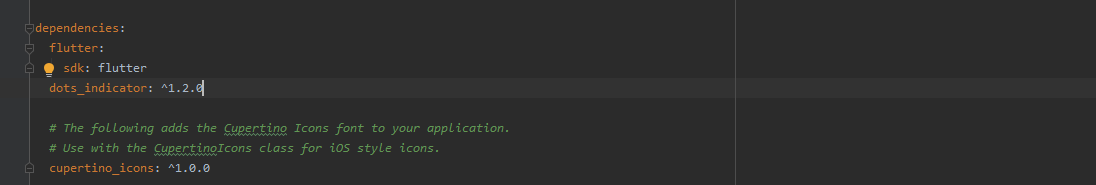GeeksforGeeks App
Open AppBrowser
Continue

# Flutter – Dots Indicator

Dots Indicator can be used to Show an increment or decrement to a value in a Flutter application through the UI. Moreover, it can also be used as an Increment or decrement component for a value through user interaction. To summarize its use case it can be improvised to use for multiple functionalities inside a flutter application.

In this article, we will look into the dots_indicator package and its uses in a flutter application by building a simple app. To build the app follow the below steps:

• Add the dependency to the pubspec.yaml file
• Import the dependency into the main.dart file
• Use StatefulWidget for structuring the application
• Initialize a state that holds a value that can be updated using buttons
• Add buttons for respective increment or decrement action

Let’s look into the steps in detail.

Use the below image as an illustration for adding the dots_indicator dependency to the pubspec.yaml file:### Importing the dependency:

To import the dependency to the main.dart file, use the below line of code:

```import 'package:dots_indicator/dots_indicator.dart';

```

### Structuring the application:

To give a simple structure to the example app, use a StatefulWidget, and extend it so that further components could be added to its body as shown below:

## Dart

 `class` `MyApp extends StatefulWidget {``  ``@override``  ``_MyAppState createState() => _MyAppState();``}`` ` `class` `_MyAppState extends State {`` ``// initialize the stage here later``  ``@override``  ``Widget build(BuildContext context) {``    ``const` `decorator = DotsDecorator(``      ``activeColor: Colors.green,``      ``activeSize: Size.square(30.0),``      ``activeShape: RoundedRectangleBorder(),``    ``);`` ` `    ``return` `MaterialApp(``      ``home: Scaffold(``        ``appBar: AppBar(``          ``title: ``const` `Text(``'GeeksForGeeks'``),``          ``backgroundColor: Colors.green,``        ``),``        ``// add contents of the body here``        ``body:``      ``)``  ``}``}`

### Initializing the State:

The state in the application can be initialized to have a default value that can be manipulated later using the buttons that we will be adding in the next step follow the below code:

## Dart

 `void` `main() => runApp(MyApp());`` ` `class` `MyApp extends StatefulWidget {``  ``@override``  ``_MyAppState createState() => _MyAppState();``}`` ` `class` `_MyAppState extends State {``  ``final _totalDots = 5;``  ``double` `_currentPosition = 0.0;`` ` `  ``double` `_validPosition(``double` `position) {``    ``if` `(position >= _totalDots) ``return` `0;``    ``if` `(position < 0) ``return` `_totalDots - 1.0;``    ``return` `position;``  ``}`` ` `  ``void` `_updatePosition(``double` `position) {``    ``setState(() => _currentPosition = _validPosition(position));``  ``}`

For the sake of simplicity, we will be adding two FloatingActionButton to increment and decrement the dots respectively based on the initial state. We will also be adding two dots indicator, one vertical and the other horizontal that will be visible in the UI as follows:

## Dart

 `FloatingActionButton(``  ``child: ``const` `Icon(Icons.``remove``),``  ``backgroundColor: Colors.green,``  ``onPressed: () {``    ``_currentPosition = _currentPosition.ceilToDouble();``    ``_updatePosition(max(--_currentPosition, 0));``  ``},``),``FloatingActionButton(``  ``child: ``const` `Icon(Icons.add),``  ``backgroundColor: Colors.green,``  ``onPressed: () {``    ``_currentPosition = _currentPosition.floorToDouble();``    ``_updatePosition(min(``      ``++_currentPosition,``      ``_totalDots.toDouble(),``    ``));``  ``},``)`

Complete Source Code:

## Dart

 `import ``'dart:math'``;`` ` `import ``'package:flutter/material.dart'``;``import ``'package:dots_indicator/dots_indicator.dart'``;`` ` `void` `main() => runApp(MyApp());`` ` `class` `MyApp extends StatefulWidget {``  ``@override``  ``_MyAppState createState() => _MyAppState();``}`` ` `class` `_MyAppState extends State {``  ``final _totalDots = 5;``  ``double` `_currentPosition = 0.0;`` ` `  ``double` `_validPosition(``double` `position) {``    ``if` `(position >= _totalDots) ``return` `0;``    ``if` `(position < 0) ``return` `_totalDots - 1.0;``    ``return` `position;``  ``}`` ` `  ``void` `_updatePosition(``double` `position) {``    ``setState(() => _currentPosition = _validPosition(position));``  ``}`` ` `  ``Widget _buildRow(List widgets) {``    ``return` `Padding(``      ``padding: ``const` `EdgeInsets.only(bottom: 20.0),``      ``child: Row(``        ``mainAxisAlignment: MainAxisAlignment.spaceAround,``        ``children: widgets,``      ``),``    ``);``  ``}`` ` `  ``String getCurrentPositionPretty() {``    ``return` `(_currentPosition + 1.0).toStringAsPrecision(2);``  ``}`` ` `  ``@override``  ``Widget build(BuildContext context) {``    ``const` `decorator = DotsDecorator(``      ``activeColor: Colors.green,``      ``activeSize: Size.square(30.0),``      ``activeShape: RoundedRectangleBorder(),``    ``);`` ` `    ``return` `MaterialApp(``      ``home: Scaffold(``        ``appBar: AppBar(``          ``title: ``const` `Text(``'GeeksForGeeks'``),``          ``backgroundColor: Colors.green,``        ``),``        ``body: Center(``          ``child: ListView(``            ``shrinkWrap: ``true``,``            ``padding: ``const` `EdgeInsets.all(16.0),``            ``children: [``              ``Text(``                ``'Current position \${getCurrentPositionPretty()} / \$_totalDots'``,``                ``style: ``const` `TextStyle(``                  ``fontWeight: FontWeight.w600,``                  ``fontSize: 16.0,``                ``),``                ``textAlign: TextAlign.center,``              ``),``              ``const` `SizedBox(height: 16.0),``              ``_buildRow([``                ``Slider(``                  ``value: _currentPosition,``                  ``max: (_totalDots - 1).toDouble(),``                  ``onChanged: _updatePosition,``                ``)``              ``]),``              ``_buildRow([``                ``FloatingActionButton(``                  ``child: ``const` `Icon(Icons.``remove``),``                  ``backgroundColor: Colors.green,``                  ``onPressed: () {``                    ``_currentPosition = _currentPosition.ceilToDouble();``                    ``_updatePosition(max(--_currentPosition, 0));``                  ``},``                ``),``                ``FloatingActionButton(``                  ``child: ``const` `Icon(Icons.add),``                  ``backgroundColor: Colors.green,``                  ``onPressed: () {``                    ``_currentPosition = _currentPosition.floorToDouble();``                    ``_updatePosition(min(``                      ``++_currentPosition,``                      ``_totalDots.toDouble(),``                    ``));``                  ``},``                ``)``              ``]),``              ``_buildRow([``                ``Text(``                  ``'Vertical'``,``                  ``style: TextStyle(fontWeight: FontWeight.w700, fontSize: 18.0),``                ``),``              ``]),``              ``_buildRow([``                ``DotsIndicator(``                  ``dotsCount: _totalDots,``                  ``position: _currentPosition,``                  ``axis: Axis.vertical,``                  ``reversed: ``true``,``                  ``decorator: decorator,``                ``),``              ``]),``              ``_buildRow([``                ``Text(``                ``'Horizontal'``,``                ``style: TextStyle(fontWeight: FontWeight.w700, fontSize: 18.0)),``                ``DotsIndicator(``                  ``dotsCount: _totalDots,``                  ``position: _currentPosition,``                  ``decorator: decorator,``                ``),``              ``]),``            ``],``          ``),``        ``),``      ``),``    ``);``  ``}``}`

Output:

My Personal Notes arrow_drop_up